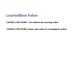# Counting Unit Cubes Volume PowerPointSubject
Resource Type
File Type
PDF (230 KB|11 pages)
\$3.00
Product Description
The PowerPoints typically include: Tiles/Links to Interactive Videos, Problem of the Day, Guided Practice, Independent Practice, and Agree or Disagree problems.

Purchase any of the other PowerPoints for Unit 4 separately or from the Unit 4 PowerPoint bundle in my store!

Unit 4 Measurement and Geometry
-Lesson 1 Line plots representing data
-Lesson 2 Interpret data using a line plot
-Lesson 3 Understand the concept of volume
-Lesson 4 Counting unit cubes volume
-Lesson 5 Use a formula to determine the volume
-Lesson 6 Find the volume of a composite figure
-Lesson 7 Convert customary units of length
-Lesson 8 Convert customary capacity
-Lesson 9 Convert customary weight
-Lesson 10 Convert metric length
-Lesson 11 Convert metric capacity
-Lesson 12 Convert metric weight
-Lesson 13 Convert units of time
-Lesson 14 Geometry quadrilaterals and polygons
-Lesson 15 Geometry triangles
Total Pages
11 pages